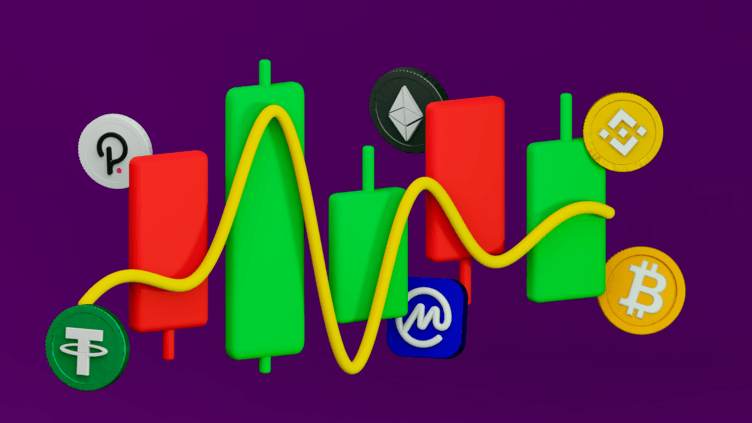Trading Insights: What Is the Relative Strength Index (RSI) in Stocks and Crypto?

# Trading Insights: What Is the Relative Strength Index (RSI) in Stocks and Crypto?

3 months ago

This week on Technical Insights, CoinMarketCap Alexandria discusses the concept of Relative Strength Index (RSI), one of the most popular technical indicators used in trading.With so many forms of technical analysis out there, sometimes it is best to go back to the basics. In today’s article, we discuss theRelative Strength Index, one of the most popular technical indicators used by traders across the board.

Chances are you have seen a price chart with a squiggly line above, and you’ve probably heard people talk about something called a divergence. This usually means the Relative Strength Index is in the mix. Let’s look at what it is, how it’s calculated, and a few examples of divergences.

## What Is the Relative Strength Index (RSI)?

The Relative Strength Index (RSI) is a so-called momentum oscillator. Momentum oscillators are technical indicators that try to visualize the strength or weakness of a certain asset. RSI moves up and down between 100 and 0. The long-standing assumption is that an asset is overbought when the RSI goes above 70 (as you can see in the picture) and is oversold when it drops below 30.

In uptrends, RSI generally stays “strong”, consolidating above the 40-50 region. When markets are weaker, downtrends are generally accompanied by RSI below 50-60.

## How Is the Relative Strength Index (RSI) Calculated?

RSI is calculated using a relatively simple formula, but explaining it fully probably goes beyond the scope of this article (and perhaps your interest). The formula uses the average bullish move and average bearish move during a certain period. Usually, this period is 14 candles. For example, in a period there were nine bullish days with an average move of 1.2%, and five bearish days with an average move of -0.7%.

These two figures can be fed into the RSI formula:

RSI =100-(100/(1+Average Bullish MoveAverage Bearish Move))

The outcome of this calculation is the RSI value for the current candle. For every new candle, the averages are re-calculated, and the RSI value changes. Now the use of RSI is not limited to looking at overbought or oversold areas. It gets interesting when the price prints a new high or low while the RSI does not. This so-called divergence between price and indicator can tell you price might see a reversal soon. Let’s look at some examples

## What Is a Relative Strength Index (RSI) bullish divergence?

A bullish divergence occurs when the RSI behaves stronger than the price itself. For example, when the price prints a lower low, but the RSI prints a higher low. This is often referred to as a strong bullish divergence, and usually signals a price reversal to the upside. The chart below shows a good example of this.

However, in addition to the above, other bullish divergences happen as well. A higher low on RSI while price makes equal lows can be seen as a bullish divergence too, or when price prints a lower low with the RSI printing equal lows. This will become clearer in the cheat sheet at the end of this article.

### What Is a Hidden Bullish Divergence?

An interesting variant of the bullish divergence is “hidden”. This divergence is slightly different because it generally signals continuation rather than reversal. A hidden divergence occurs when the RSI makes a lower low, whereas the price makes a higher low. This gives the RSI more room to run, whereas the price remains strong. The chart below is a recent example of a hidden bullish divergence resulting in continuation.

The above chart is a scenario of the price and RSI diverging in a bullish manner. As you can tell, bullish divergences always happen on lows, both in price and RSI. Let’s look at the bearish version of these examples.

## What Is an RSI Bearish Divergence?

A bearish divergence occurs when the RSI is weaker than the price. For example, when the price hits a higher high, but the RSI prints a lower high. This is often referred to as a strong bearish divergence and usually signals a price reversal to the downside. The chart below shows a good example of this.

There are other types of bearish divergences too, though! A lower high on RSI while price makes equal highs is bearish too. Another scenario is when the price prints a higher high while RSI prints equal highs. Again, there’s a cheat sheet at the end of this article to visualise these divergences.

### What Is a Hidden Bearish Divergence?

Like its bullish counterpart, the bearish divergence also has a hidden variant. It works similarly in the sense that this divergence too generally signals continuation rather than reversal. A hidden bearish divergence occurs when the RSI makes a higher high, whereas the price makes a lower high. This gives the RSI more room to fall, whereas the price remains weak. The chart below is a great example of this.

There are a few examples of price and RSI showing bearish divergences. As you can tell, bearish divergences happen on the highs, both in price and RSI.

## ​​Divergence Cheat Sheet

Before we reach the end, I’ve cooked up a little cheat sheet to visualize the examples I gave along the way. It shows the strong, medium, and even the weakest divergences. But the hidden divergences - well, let’s just say they’re hiding!

## Closing Statements

As with any other trade signal, I like to combine divergences with support and resistance. When you see a bearish divergence shaping up into resistance, you have a strong confluence to enter a short trade. These are just a few simple ways to get started with the relative strength index. There are more complex ways of analyzing charts since you can take RSI as far as you want.